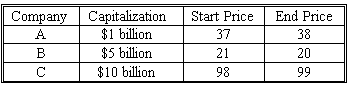### CFA Practice Question

There are 275 practice questions for this study session.

### CFA Practice Question

The Board of Governors of the Nottingham Share Exchange wishes to publish an index using all three issues currently listed. They want to know how index performance might be affected by the choice of a price-weighted, a value-weighted, or an unweighted index. Advise them how the index would have changed on February 18, a day when no splits occurred, based upon the following data:A. Price-Weighted and Value-Weighted Indices Up, Unweighted Index Unchanged
B. Price-Weighted Index Up, Value-Weighted and Unweighted Indices Down
C. Price-Weighted Index Up, Value-Weighted Index Down, Unweighted Index Up
Explanation: Price-weighted index = Sum of prices / an adjusted divisor
Since there were no splits, the divisor is constant.
(38 + 20 + 99) > (37 + 21 + 98)
157 > 156
A price-weighted index would have gone up by 0.6%.

Value-weighted index = Beginning Value x (Ending index capitalization) / (Beginning index capitalization)
For any beginning value, the daily change = ending capitalization / beginning capitalization.
1.00 x (38 / 37) + 5.00 x (20 / 21) + 10.00 x (99 / 98) < 1.00 + 5.00 + 10.00
1.027 + 4.762 + 10.12 < 16.000
15.891 < 16.000
A value-weighted index would have gone down by 0.7%.

An unweighted index is based upon the arithmetic average of percent changes for each stock.
[(38 / 37 - 1) + (20 / 21 - 1) + (99 / 98 - 1)] / 3 = [2.7% + (-4.7%) + 1.0%] / 3
= -1.0% / 3
An unweighted index would have gone down by 0.3%.